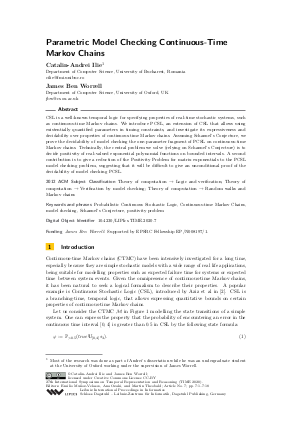Document# Parametric Model Checking Continuous-Time Markov Chains

### Authors Catalin-Andrei Ilie, James Ben Worrell## File

LIPIcs.TIME.2020.7.pdf
• Filesize: 0.54 MB
• 18 pages

## Cite As

Catalin-Andrei Ilie and James Ben Worrell. Parametric Model Checking Continuous-Time Markov Chains. In 27th International Symposium on Temporal Representation and Reasoning (TIME 2020). Leibniz International Proceedings in Informatics (LIPIcs), Volume 178, pp. 7:1-7:18, Schloss Dagstuhl - Leibniz-Zentrum für Informatik (2020)
https://doi.org/10.4230/LIPIcs.TIME.2020.7

## Abstract

CSL is a well-known temporal logic for specifying properties of real-time stochastic systems, such as continuous-time Markov chains. We introduce PCSL, an extension of CSL that allows using existentially quantified parameters in timing constraints, and investigate its expressiveness and decidability over properties of continuous-time Markov chains. Assuming Schanuel’s Conjecture, we prove the decidability of model checking the one-parameter fragment of PCSL on continuous-time Markov chains. Technically, the central problem we solve (relying on Schanuel’s Conjecture) is to decide positivity of real-valued exponential polynomial functions on bounded intervals. A second contribution is to give a reduction of the Positivity Problem for matrix exponentials to the PCSL model checking problem, suggesting that it will be difficult to give an unconditional proof of the decidability of model checking PCSL.

## Subject Classification

##### ACM Subject Classification
• Theory of computation → Logic and verification
• Theory of computation → Verification by model checking
• Theory of computation → Random walks and Markov chains
##### Keywords
• Probabilistic Continuous Stochastic Logic
• Continuous-time Markov Chains
• model checking
• Schanuel’s Conjecture
• positivity problem

## Metrics

• Access Statistics
• Total Accesses (updated on a weekly basis)
0

## References

1. S Akshay, Timos Antonopoulos, Joël Ouaknine, and James Worrell. Reachability problems for Markov chains. Information Processing Letters, 115(2):155-158, 2015.2. Adnan Aziz, Kumud Sanwal, Vigyan Singhal, and Robert Brayton. Model-checking continuous-time Markov chains. ACM Transactions on Computational Logic (TOCL), 1(1):162-170, 2000.3. Paul Bell, Jean-Charles Delvenne, Raphael Jungers, and Vincent D. Blondel. The continuous Skolem-Pisot problem: On the complexity of reachability for linear ordinary differential equations. Theoretical Computer Science, 411(40–42):3625-3634, 2010.4. Ventsislav Chonev, Joel Ouaknine, and James Worrell. On the Skolem problem for continuous linear dynamical systems. 43rd International Colloquium on Automata, Languages, and Programming (ICALP), pages 100:1-100:13, 2016.5. Henri Cohen. A Course in Computational Algebraic Number Theory. Springer, 1993.6. Paul M. Cohn. Basic Algebra: Groups, Rings and Fields. Springer, second edition, 2004.7. Bettina Just. Integer relations among algebraic numbers. In International Symposium on Mathematical Foundations of Computer Science, pages 314-320. Springer, 1989.8. Marta Kwiatkowska, Gethin Norman, and David Parker. PRISM 4.0: Verification of probabilistic real-time systems. Proc. 23rd International Conference on Computer Aided Verification (CAV’11), volume 6806 of LNCS, pages 585-591, Springer, 2011.9. Angus Macintyre and Alex J Wilkie. On the decidability of the real exponential field. In (ed. Piergiorgio Odifreddi) Kreiseliana: About and Around Georg Kreisel., 1996.10. Ivan Niven. Irrational Numbers. The Mathematical Association of America, fifth edition, 2005.11. Paul S Wang. Factoring multivariate polynomials over algebraic number fields. Mathematics of Computation, 30(134):324-336, 1976.12. Boris Zilber. Exponential sums equations and the Schanuel conjecture. Journal of the London Mathematical Society, 65(1):27-44, 2002.X

Feedback for Dagstuhl Publishing